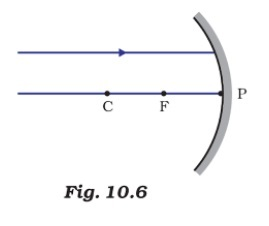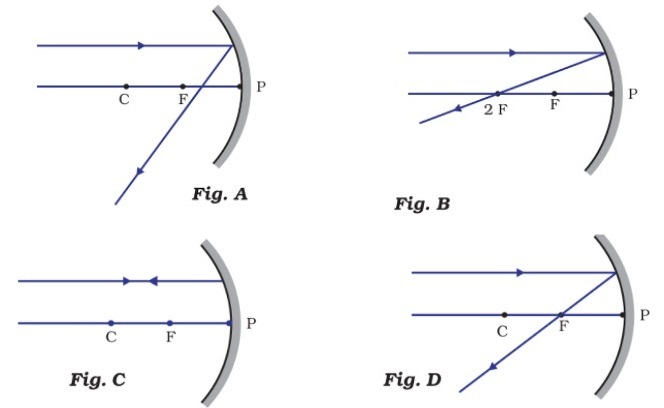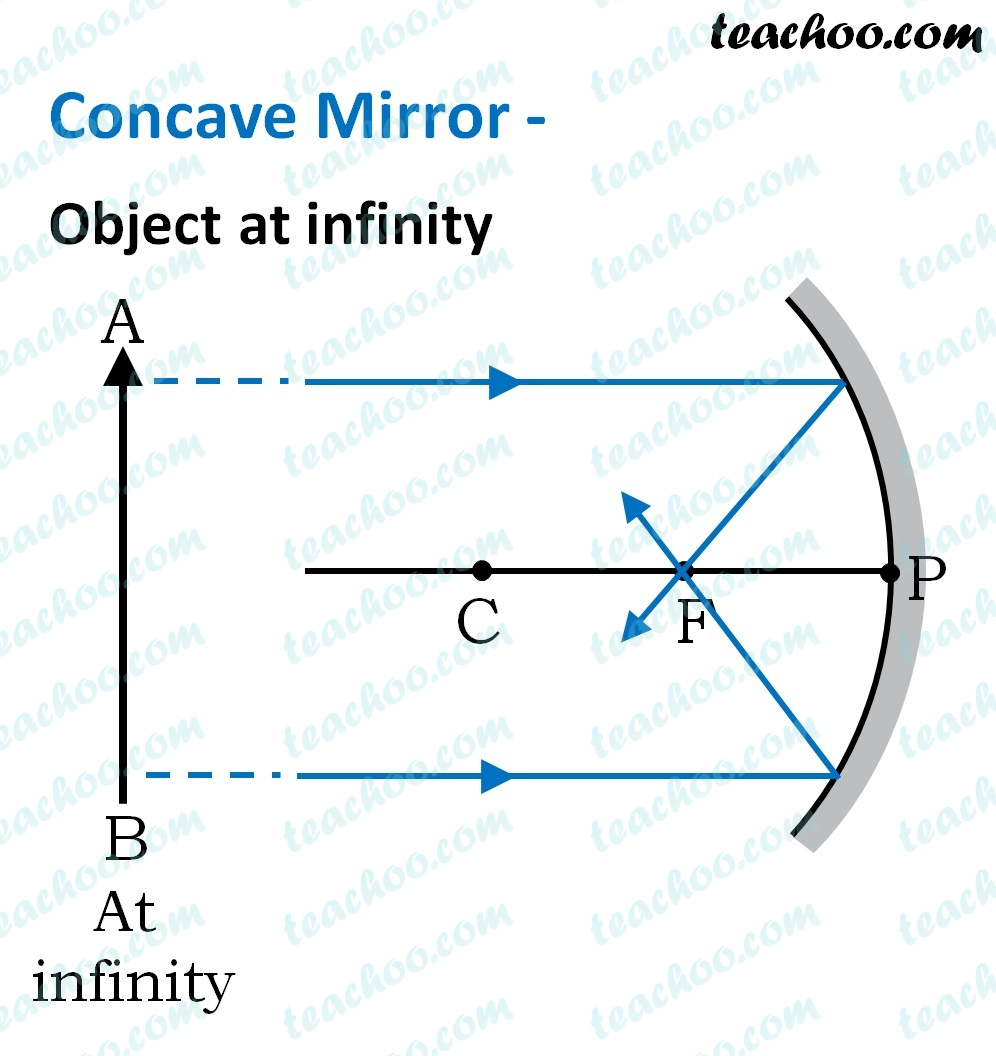MCQs from NCERT Exemplar

Class 10
Chapter 10 Class 10 - Light - Reflection and Refraction (Term 1)

## Which of the following ray diagrams is correct for the ray of light incident on a concave mirror as shown in Figure 10.6?## (d) Fig. DThe figure shown is that of a concave mirror .

A concave mirror reflects parallel light and converges it at the focus .Since in only Figure D the incident ray converges at focus.

So, the correct answer is (d)